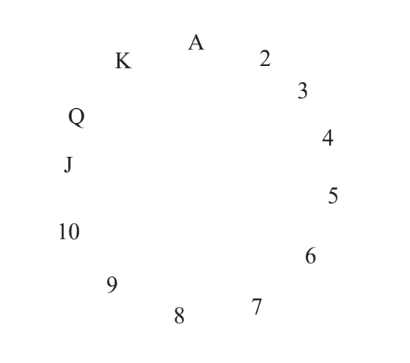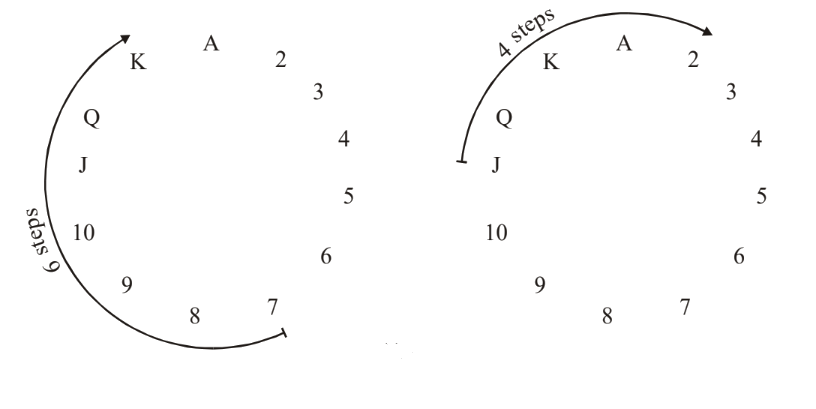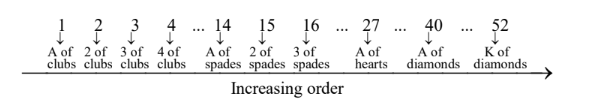# Permutations and Combinations Set 6

Go back to  'SOLVED EXAMPLES'

Example – 36

Prove that for $$n \ge 4,$$  the sum $$1!\, + \,2!\, + \,3!\, + ... + \,n!$$ cannot be the square of a positive integer.

Solution: The approach lies in realizing the general properties of a perfect square. Note that a square will have, at its unit place, a digit from amongst the set {1, 4, 5, 6, 9}. It cannot have a ‘3’ or an ‘8’ as its unit digit. Work it out yourself.

Now, for the given sum, the first four terms add to 33:

$1! + 2! + 3! + 4! = 1 + 2 + 6 + 24\\ \;\;\;\;\;\;\;= 33$

For any integer greater than 4, its factorial will always have a ‘0’ at its unit place. Can you see why?

This means that the given sum will have a ‘3’ at its unit place, so it cannot be a perfect square!

Example – 37

A set P contains x elements while Q is another set which contains y elements. How many functions  $$f:P \to Q$$ exist which are (a) one - one (b) onto?

Solution: Obviously, the total number of functions possible is $${y^x}$$

(a) If the function is to be one-one, y, the number of elements in the co-domain of f, must be greater than or equal to x :

$y \ge x$

In that case, the number of one-one functions will simply be $${}^y{P_x},$$   since you are creating a mapping from x elements in P to x elements in Q, out of a total y elements in Q.

(b)   For the function to be onto, the only constraint that needs to be satisfied is that each element in the co-domain Q must have a pre-image, which means that x must be greater than or equal to y.

The remaining part is left to the reader as an exercise.

Example – 38

Four non-identical dice are rolled simultaneously. How many rolls are possible in which 6 shows up on at least  one dice?

Solution: Its easiest to proceed by considering the complementary case:

Total number of rolls possible  :  6 4      (Why ?)

Rolls in which no 6 shows up   :  5 4     (Why ?)

Our answer is therefor 6 4 – 54.

Now, a very important issue which we leave to you to resolve: what if the four dice were identical?

Example – 39

In an examination, the maximum marks for each of three papers is n and that for the fourth paper is 2n. Find the number of ways in which a candidate can get 3n marks.

Solution: We need to find the number of solutions to the non-negative integral solution

${x_1} + {x_2} + {x_3} + {x_4} = 3n,...{\rm{ }}\left( 1 \right)$

Where $${x_1},\,{x_2},\,{x_3}$$   all lie in [0, n], while $${x_4}$$  can vary upto   $$2n,$$  i.e., $${x_4} \in [0,\,2n],$$  where all the $$x_i^′\,s$$   are integers. Let us construct the polynomial  $$P(x)$$ given by

$P(x) = {(1 + x + {x^2} + ... + {x^n})^3}(1 + x + x + ... + {x^{2n}})$

Now, think hard ! The first bracket in  $$P(x)$$ is actually three identical brackets $$(1 + x + {x^2} + ... + {x^n}).$$

When   $$P(x)$$  is expanded , each of these three brackets can contribute at the most an n th power in x, whereas the fourth bracket can contribute upto  2n in powers of x.

When  $$P(x)$$ is expanded and the terms written out, note that $${x^{3n}}$$  will also be formed. But what will be the coefficient of $${x^{3n}}\,?$$ It will be precisely the number of solutions to the equation (1) under   consideration !

Why ? Because, each time  $${x^{3n}}$$ is formed is a unique combination of power contribution from each of the four brackets. For example,

$P(x) = \underbrace {(\mathop {1 + x + ...\,{x^n}}\limits_{\scriptstyle\atop{\scriptstyle\,\,\,\,\,\, \downarrow \atop\scriptstyle{\rm{Take 1}}}} )(\mathop {1 + x + ...\,{x^n}}\limits_{\scriptstyle\atop{\scriptstyle\,\,\,\,\,\,\, \downarrow \atop\scriptstyle{\rm{Take 1}}}} )(\mathop {1 + x + ...\, + {x^n}}\limits_{\scriptstyle\atop{\scriptstyle\,\,\,\,\,\,\,\, \downarrow \atop\scriptstyle{\rm{Take }}{x^n}}} )(\mathop {1 + x + ... + {x^{2n}}}\limits_{\scriptstyle\,\,\,\,\,\,\,\,\atop{\scriptstyle\,\,\,\,\,\,\, \downarrow \atop\scriptstyle{\rm{Take }}{x^{2n}}}} )}_{{\rm{Product formed = }}{x^{3n}}}$

In this example, we take the term 1( or $${x^0}$$) from the first two brackets, $${x^n}$$  from the third and $${x^{2n}}$$  from the fourth. This is a unique combination in which $${x^{3n}}$$   can be generated, but this is only one such. There will be many more ways in which $${x^{3n}}$$ can be generated. How many ? Precisely, the coefficient of  $${x^{3n}}$$ when $$P(x)$$  is expanded !

To find out the coefficient, you’ll have to wait until you are familiar with the Binomial / Multinomial expansion.

Example – 40

In how many ways can one fill an m × n table with  $$\pm 1$$ such that the product of the entries in each row and each column equals –1?

Solution:    Denote by  $${a_{ij}}$$ the entries in the table. For $$1 \le i \le m - 1$$ and $$1 \le j \le n - 1,$$     we let  $${a_{ij}} = \pm 1$$ in an arbitrary way. This can be done in $${2^{(m - 1)(n - 1)}}$$   ways. The values for $${a_{mj}}with\;1 \le j \le n - 1,$$  and for  $${a_{in}}with\;1 \le i \le m - 1,$$ with  are uniquely determined by the condition that the product of the entries in each row and each column equals –1. The value of    $${a_{mn}}$$ is also uniquely determined, but it is necessary that

$\prod\limits_{j = 1}^{n - 1} {{a_{mj}}} = \prod\limits_{i = 1}^{m - 1} {{a_{in}}} \cdot \left( * \right)$

If we denote by

$P = \prod\limits_{i = 1}^{m - 1} {\prod\limits_{j = 1}^{n - 1} {{a_{ij}}} }$

we observe that

$P\prod\limits_{j = 1}^{n - 1} {{a_{mj}} = {{( - 1)}^{n - 1}}}$

And

$P\prod\limits_{i = 1}^{m - 1} {{a_{in}} = {{( - 1)}^{m - 1}}}$

Hence (*) holds if and only if m and n have the same parity.

## CONCLUSION :    A MAGIC TRICK

For those of you interested in cards, here is a very interesting card trick that has its basis in an elementary application of this chapter’s concepts.

A magician sends out his assistant into the audience with a well-shuffled standard deck of cards. The assistant asks 5 different people chosen at random from this audience to pick a card each from the deck. The magician is     on-stage and cannot see the cards drawn from the deck. The magician then asks the assistant to reveal 4 of the 5 drawn cards while keeping the fifth card secret.

The magician then thinks for a while and tells the audience the number and suit on the fifth secret card ! We have to figure exactly how the assistant conveys the information about the fifth card to the magician. You can assume that no one is cheating!

The trick explained

Somehow, the assistant has been able to convey information about the fifth card to the magician, even though he reveals only 4 of the 5 cards. The information must be embedded in the order in which the assistant reveals the 4 cards. Thus, the magician and assistant must have pre-decided on a scheme whereby the magician can deduce the secret card from the order of the 4 revealed cards. Here is how this can be done.

Since 5 cards are drawn, at least 2 cards must be of the same suit. If there are more than 2 cards of the same suit, the assistant simply chooses any 2 of them.

Now, one of these 2 cards will be revealed first by the assistant while the other card will become the secret card. So, the magician knows from the suit of the first revealed card what the suit of the secret card is.

The first issue is that, of the 2 cards of the same suit, how does the assistant decide which one to reveal and which one to keep secret?

For that, the assistant refers to the following diagram (mentally of course!):Fig. 17

In this circular arrangement, observe that the difference between any two members is at the most 6. For example, the difference between “7” and “Q” is 6 while that between “J” and “2” is 4Fig. 18

Thus, of the 2 cards of the same suit, the assistant reveals that card from which it is possible to reach the other card in at most 6 steps.

For example, if the assistant finds a “J” of Hearts and a “2” of Hearts in the 5 drawn cards, he will reveal the “J” of Hearts first and keep the “2” of Hearts secret since in our circular arrangement it is possible to reach from “J” to “2” in 4 steps.

Thus, till now the scheme is that the secret card is of the same suit as the first revealed card and lies within at the most 6 steps from the first card, that is, the offset of the secret card from the first card is at the most 6.

We now require a scheme whereby the assistant can communicate the offset to the magician.

For this purpose, they pre-decide upon an order of all the 52 cards of the deck. For example, suppose they order the deck like follows:Fig. 19

Now, after the assistant has revealed the first card, he has 3 more cards to reveal. Those 3 cards can be ordered according to the order of Fig 19. Thus, one of those 3 cards will be the ‘smallest’, one will be the ‘middle’ card and one will be the ‘largest’. Label the 3 cards as S, M and L.

To communicate the offset to the magician, the assistant reveals the 3 cards in a particular order as shown in the table below

 Offset Order in which the assistant reveals the 3 cards 1 SML 2 SLM 3 MSL 4 MLS 5 LSM 6 LMS

Since the maximum offset can be 6, the 6 permutations of S, M and L cover all the possible offsets!

Once the offset is communicated, the magician simply adds it to the first card to deduce the fifth, secret card!

Let us try this out with a particular example. Suppose that the following 5 cards are drawn: “A of spades” “2 of Hearts” “3 of clubs” “7 of Hearts” “8 of clubs”.

The assistant picks 2 cards of the same suit. Here we have 2 options for the 2 cards. We can choose either. Suppose the assistant chooses “2 of Hearts” and “7 of Hearts”. The offset of “7” from “2” is 5 which is less          than 6.

Therefore, “2 of Hearts” is the first card that the assistant reveals.

To communicate the offset, the assistant orders the three other cards (to be revealed) according to Fig. 19 as follows:

$\begin{matrix} ''\text{3 of clubs}'' & < & ''8\text{ of clubs}'' & < & ''\text{A of spades}'' \\ \text{S} & {} & \text{M} & {} & \text{L} \\ \end{matrix}$

Since the offset required is 5, the assistant reveals these three cards (according to the Table on the previous page) in the order L S M.

Thus, this is what the assistant says:

“2 of Hearts”